# Three Ways to Use Ten Frames for Upper Elementary {Powerful!}Ten frames are a staple in primary classrooms. These two-by-five rectangles can be used to help young students develop number sense within ten. But their uses extend far beyond basic addition and subtraction! Here are three ways that upper elementary students can benefit from using ten frames in the math classroom.

## Using Ten Frames to Reinforce the Base 10 System

In our base ten number system, each place value is ten times greater than the place value to its right. Ten frames can be used to model this abstract math concept.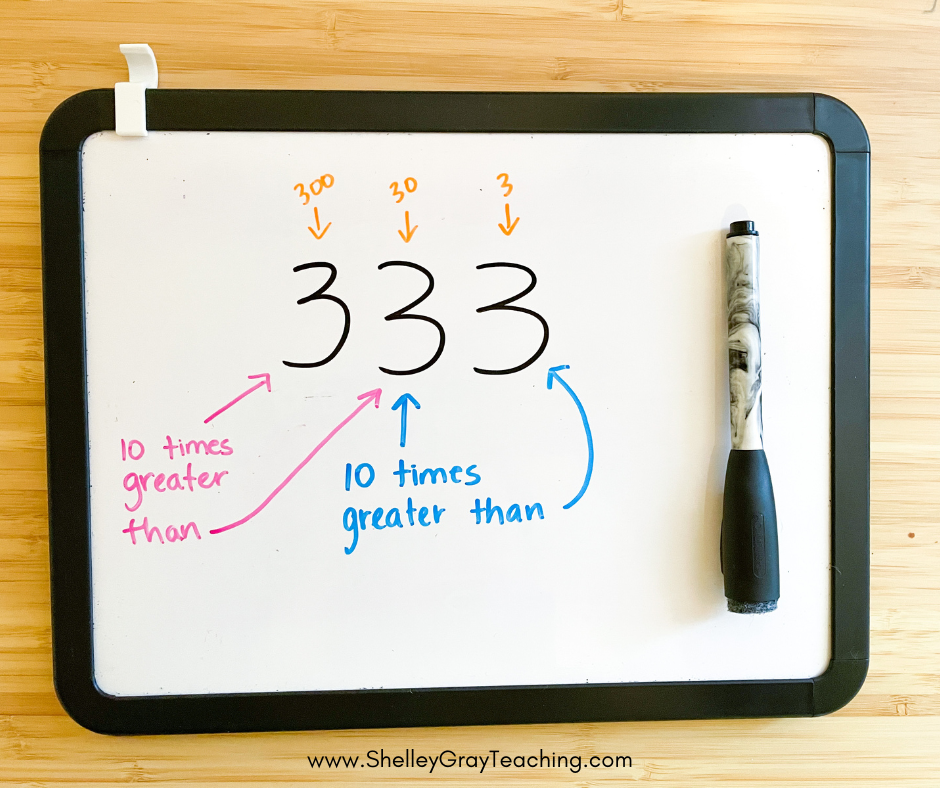For this activity, you will need a ten frame and place value tokens. If you don’t have place value tokens, you can easily have students make them. For the ones in the picture below, I used a dry erase marker to write on the ten frame dots that came with this set.

Begin by modeling a digit in the ones place. For example, to model the number 3, a ones place token would be placed in three of the boxes on the ten frame.To make a number ten times greater, exchange the ones place tokens for the tens place tokens. By making the place value ten times greater, the new number represented is 30.Continue by exchanging the tens place tokens with hundreds place tokens (creating 300), and so on.## Using Ten Frames to Model Multiplication and Division

While ten frames are most commonly used to teach addition and subtraction, they are also incredibly useful for multiplication and division!

For this activity, you will need a ten frame. You can also use either a dry erase marker, counters, or dice. You will be modeling that multiplication is repeated addition- or adding up equal groups. Similarly, division is repeated subtraction, or splitting a number into equal groups.

For multiplication, model a problem such as 4 x 5. This can be expressed as “four groups of 5.” Have students model this expression on their ten frames. Ask students to develop strategies for finding the product. This could include skip counting as they point to each box on the frame. They may also be able to visualize other strategies, such as adding two groups of five plus two groups of five.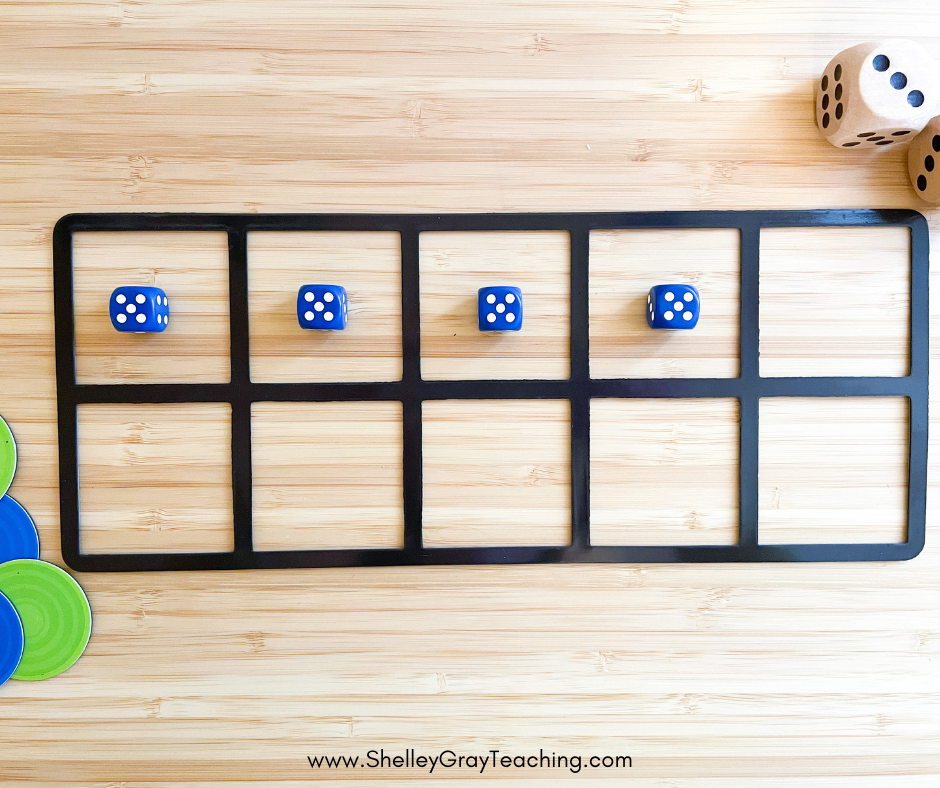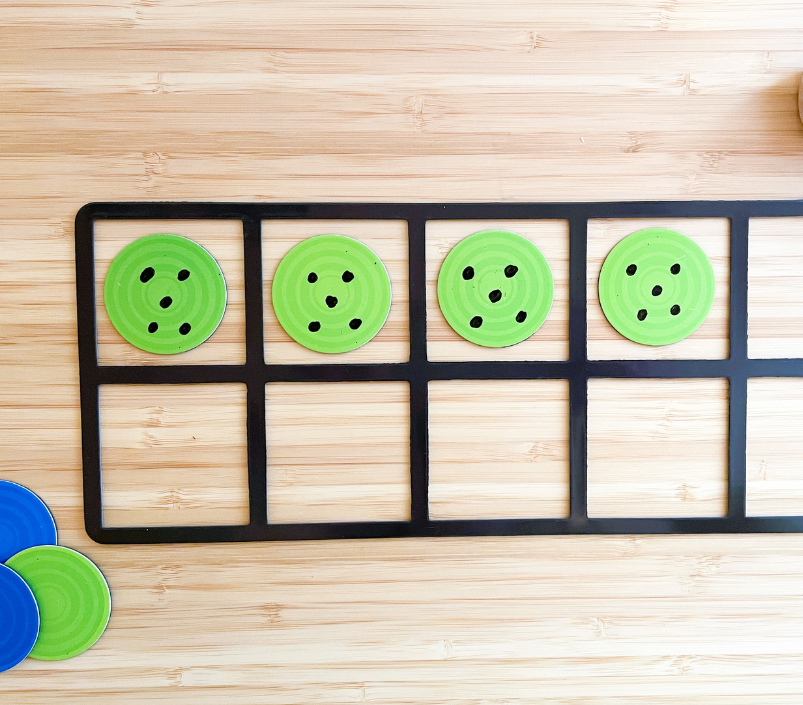For division, students begin with the total number of counters. For example, when working with the expression 20 ÷ 5, students will each get 20 counters. Now, this expression can be interpreted as 20 divided evenly among 5 groups, or 20 divided into groups of 5. Students can explore both these interpretations on their tens frame to find the quotient.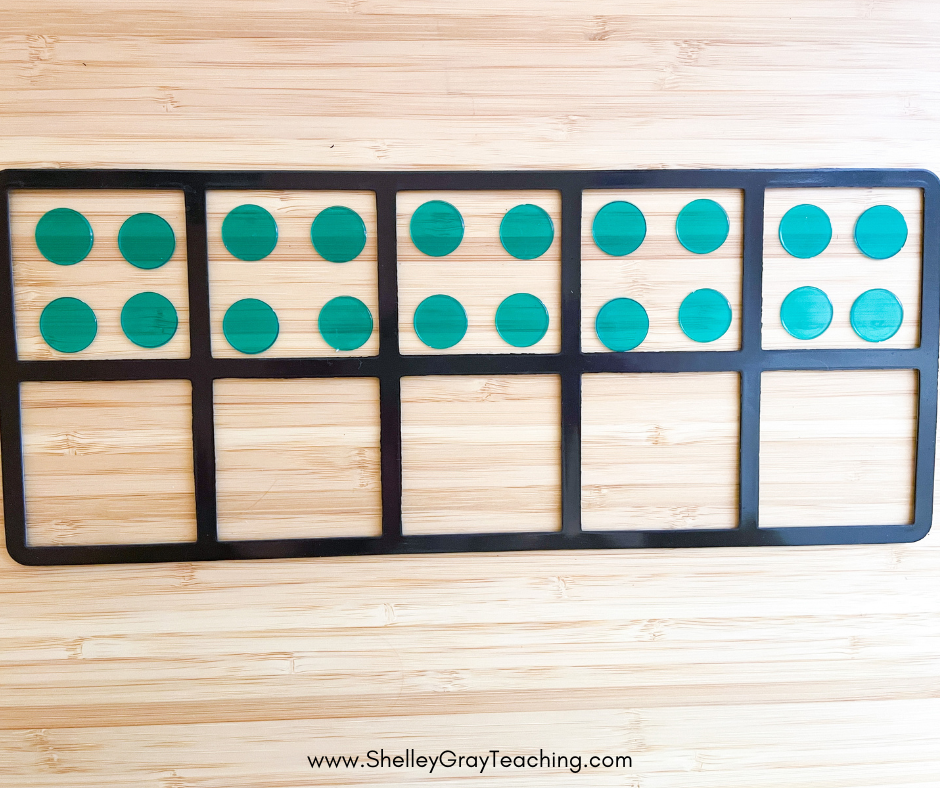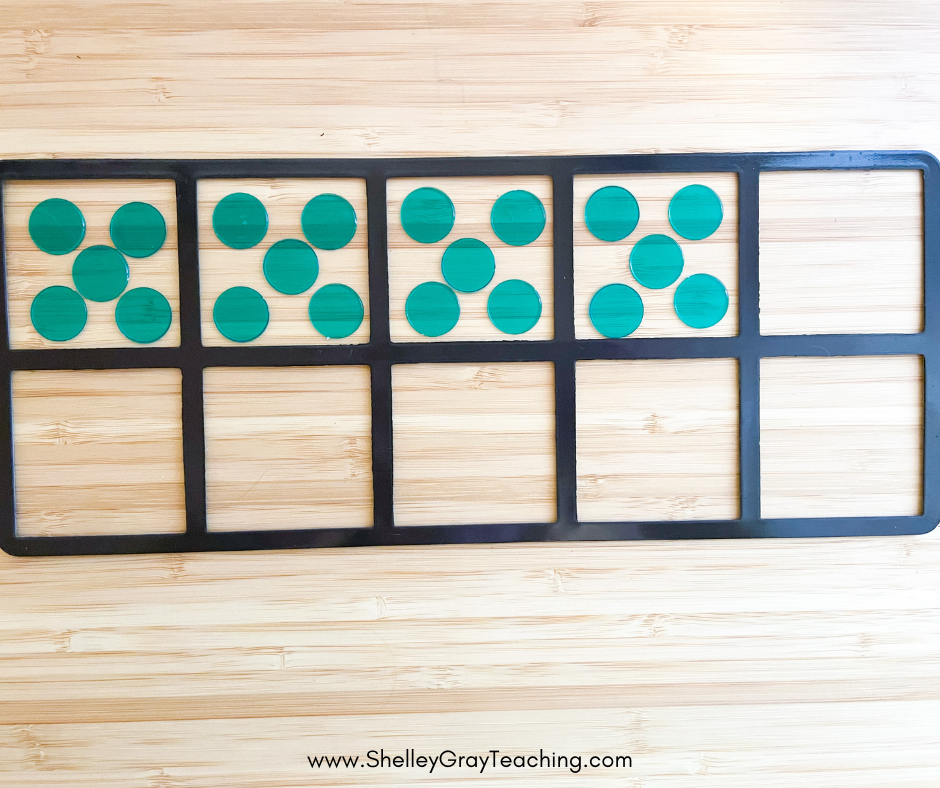Looking for ways to incorporate real-life into your math class? Real -life math projects show your students how math is used in the real world, incorporating relevant, interesting topics! Get your kids hooked on math projects with a FREE Camping Trip Math Project HERE

## Developing Computation Strategies

As students get older, they encounter longer and more complex mathematical situations. Developing computational fluency with addition and subtraction is more important than ever. When students struggle with computation errors it can be useful to get back to the basics and spend some time “seeing the math” inside a ten frame.

Designate each addend as a separate color. In this example, you see that 7 is green, while 5 is blue. Have the student model each addend in a separate ten frame. Then, use the ten frames to introduce new strategies for addition.For example, if the student is still counting on to add, try a make ten strategy. Take three blue counters from 5 and use them to fill the frame that shows 7. Now, instead of adding 7 and 5, we are adding 10 and 2.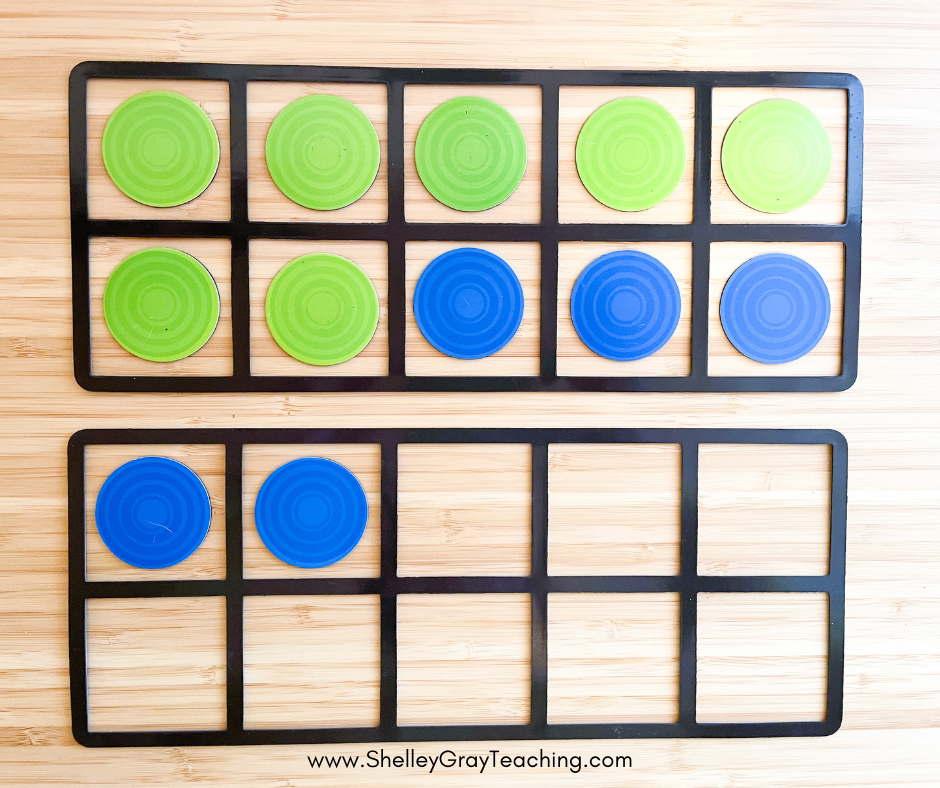We can extend this same idea to an expression like 70+50 by using our place value tokens again. In the first picture, we see 70+50 modelled with each addend in a separate ten frame. In the second picture, we took 30 from the 50 to make 100. Now we are working with an easier expression – 100+20.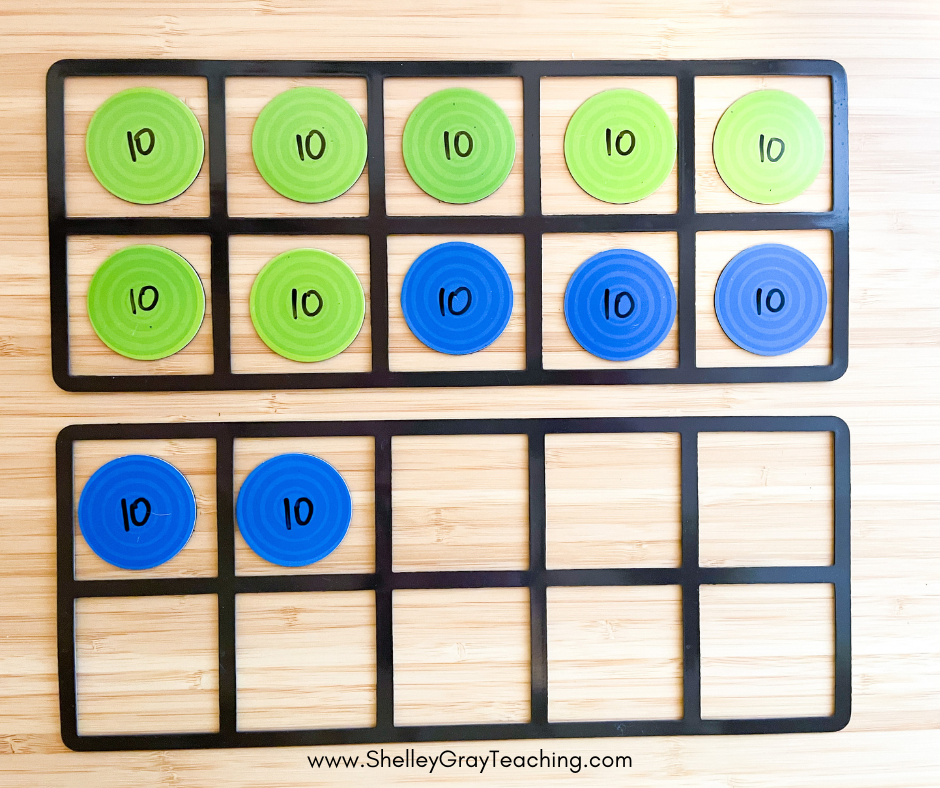Another strategy that can be modeled with ten frames is creating doubles. By moving one green counter to the second ten frame, the problem changes from 70 + 50 to 60 + 60. With repeated exposure to these manipulatives, students will internalize these flexible strategies. This will lead to more successful computation within their grade level problem-solving!It’s time to think of ten frames as more than just a tool for primary grades. They can help our intermediate students make connections with abstract math concepts and model new strategies!

This site uses Akismet to reduce spam. Learn how your comment data is processed.